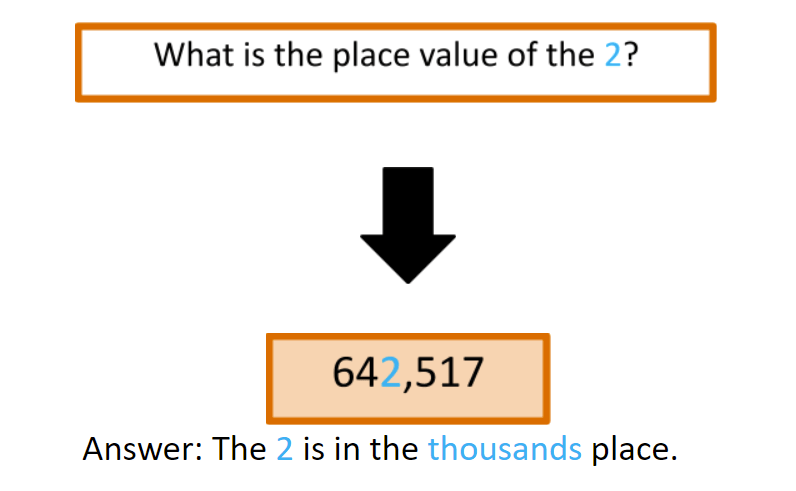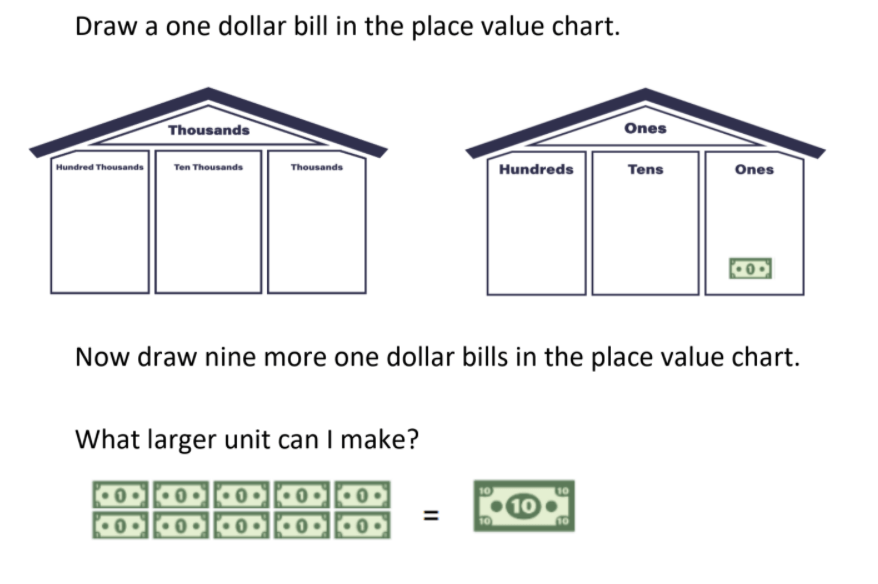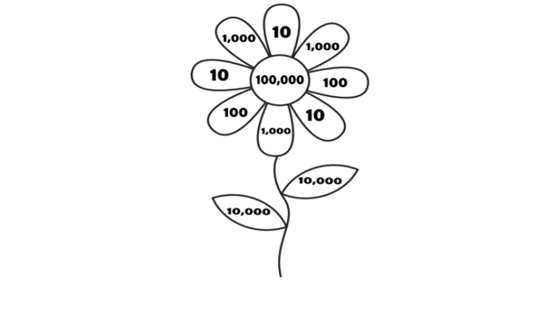# How to Teach Place Value to 4th Graders

 Listen to this Lesson:

Place value is arguably one of the most important math concepts in the curricula of elementary school. As early as grade one, children start to recognize place value in numerals beyond 20.

By grade four, students should have developed a solid understanding of place value in higher numbers, including three-digit numbers. Thus, they should be well-prepared to tackle the four-digit ones and solve complex math problems by using their place value knowledge.

But as a 4th-grade math teacher or a homeschooling parent, you’ll also need to be well-prepared to support your students to further develop their place value knowledge and reinforce previous knowledge.

Check out these simple tips on how to teach place value to 4th graders that will help your students breeze through this math concept!

## What Is Place Value?

You may want to start your lesson by polishing up on previously acquired knowledge about place value. For instance, explain what place value is and add a few simple examples.

Place value is simply the value of each digit in a given number, or the value of where a digit is in a number.

For example, in 453, the 5 is in the ‘tens’ place, so its place value is 10.

In 571, the 5 is in the ‘hundreds’ place, so its place value is 100.

In 5 386, the 5 is in the ‘thousands’ place’, so its place value is 1000.

In other words, by understanding place value, we recognize that while a number can be the same, its value will depend on the place or position where the digit is in a specific number.

Consider also using this video by Khan academy to provide a nice visual explanation of finding a number’s place value.## Why Is Place Value Important?

Your students might be wondering why they should be learning about place value at all. This is not infrequent among math students in any grade and should be tackled accordingly.

One way to simplify the importance of place value to young learners is to tell them that by understanding it, they understand the difference between the \$75 they received as a birthday gift from grandpa and the \$786 on the tag of that iPad they want to save up for.

You could also add that the concept of place value lays the foundation for regrouping, multiplication of multiple-digit numbers, and a plethora of other operations in the decimal system and beyond. Understanding place value is essential for later arithmetic and algebra learning.In short, almost all mathematical notions and a variety of practical experiences from daily life build on place value – that’s why it’s so important to understand it.

### Place Value Makes Math Easier

Point out the importance of place value by explaining to students that the place value to the left of the digit is 10 times bigger compared to the digit on the right, which also implies that the place value to the right of the digit is 10 times smaller compared to the digit to its left (ex: 100 ÷ 10 = 10).

To demonstrate to kids how this principle facilitates things for them, you can use several math problems, for instance:

20×50 = 1,000 because we know that 5×2 = 10 and then we simply add the two zeros that we were left with.

300÷50 = 60 because we know that 30÷5 = 6 and then we simply add the zero that we were left with.

## Activities to Teach Place Value to 4th Graders

Now that you’ve reviewed the definition of place value and its importance, you may proceed with more practical activities and exercises that will reinforce this knowledge.

But first, you may consider starting with a warm-up activity on group counting.

### Group Counting in Tens

If you notice that some of the students are still struggling with place value as a concept, you may want to train the class to do group counting.

If children are able to chunk together groups of small numbers into big numbers, you’re setting the foundation for them to break down larger numbers into place values.

So, introduce a brief activity on group counting. Bring some marbles (around 100) to class. Just make sure they’re more or less homogenous. Ask children to stand in a circle. Now scatter the marbles in the middle of the circle and tell children to find the easiest way to count the marbles.

Some students may come up with a solution on their own, and if they don’t, give them a hint that in modern math and in our numeration system, the number 10 is used as a base. Encourage them to count in groups of 10.

Children will be able to realize that grouping ten marbles into ten groups makes counting easier than counting marbles one by one.

### Place Value Flower Puzzle

Print out the flower puzzle of this place value assignment (page 1) and create a fun, coloring activity in your class to practice place value! You’ll need crayons in five different colors (yellow, red, green, orange, and purple). Make sure you have enough crayons for all children.

Now distribute the worksheets and ask students to solve the math problems in the assignment and present the answers by coloring the answer in the appropriate color.

That is, if the math problem is for example 50 x 20,000, the student should first calculate the answer to this by relying on their place value knowledge. Since the answer to this math problem is 100,000, they should color one flower petal on which the number 100,000 is written.

Tens should be colored yellow, hundreds are red, thousands are green, ten thousands are orange, and hundred thousands are purple.

Once students finish the activity, ask them to present their colorful flowers and explain their reasoning process of how they solved the math problems by making references to place value.### Place Value Chart

Another activity to teach place value to 4th graders involves using a place value chart. Use a place value chart with different place values, such as this awesome house-shaped place value chart (page 3), or you can create your own chart. Use ones, tens, hundreds, thousands, ten thousands, and hundred thousands as place value columns.

Print out a copy for each student and assign several multi-digit numbers for children to put them inside the house chart, with each digit in the appropriate place value column. You can start by giving children an example. For instance, show them how to put the number 456,783 in the chart.

Explain that the 4 should be out in the hundred thousands value, the 5 in the ten thousands value, the 6 in the thousands value, the 7 in the hundreds value, the 8 in the tens value, and the 3 in the ones value.

After giving them an example of how to complete this activity, provide different multi-digit numbers and let them practice until they get the hang of it.

### Place Value Quiz

Create a small quiz game to practice place value. You can use the questions from this place value quiz and expand them further by adding a few more math problems, depending on how long you want the quiz to last.

Divide children into groups of 3, 4. Print out the quiz questions and distribute them to each group. If you decide to expand the math problems, feel free to can include more questions such as:

• What is the place value of the underlined digit in 876 463?
• What is the place value of the underlined digit in 9 348 210?
• Calculate the answer to 12 000 ÷ 30 by using place value

## Before You Leave…

We hope you’ve enjoyed our tips on teaching math! Make sure to check out our worksheets and resources on how to teach place value to 4th graders. These are all pdf files, so you’ll be able to print them out easily:

To get the Editable versions of these files, join us inside the Math Teacher Coach Community:

These activities are from:

Unit 1 – Place Value and Rounding of Multi-Digit Whole Numbers

### Do You Need More Math Resources?

If you’re looking for more math materials for kids of all ages, sign up for a membership on MathTeacherCoach or head over to our blog – you’ll find that with the resources we offer, teaching math has never been easier!